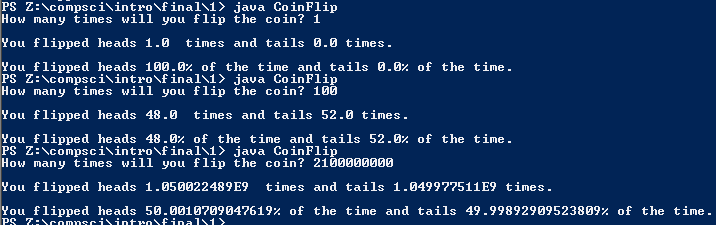# Final Exam 1

## Code

```      /// Name: Alex Pinder
/// Period: 5
/// Program Name: Final Semester 1
/// File Name: CoinFlip.java
/// Date Complete: 1/20/16

import java.util.Random;
import java.util.Scanner;

public class CoinFlip
{
public static void main ( String[] args )
{
Scanner keyboard = new Scanner(System.in);
Random r = new Random();

int times;

do
{
System.out.print( "How many times will you flip the coin? " );
times = keyboard.nextInt();

if ( times <= 0 )
System.out.println( "Choose a valid number" );
if ( times > 2100000000 )
System.out.println( "Choose a valid number" );
} while ( times <= 0 || times > 2100000000 )

int flip, counter;

timesTails = 0;
counter = 0;

while ( counter != times )// This doesn't actually have to be a do-while loop so i didn't use one.
{
flip = 1 + r.nextInt(2);

if ( flip == 1 )

else if ( flip == 2 )
timesTails++;

counter++;

}